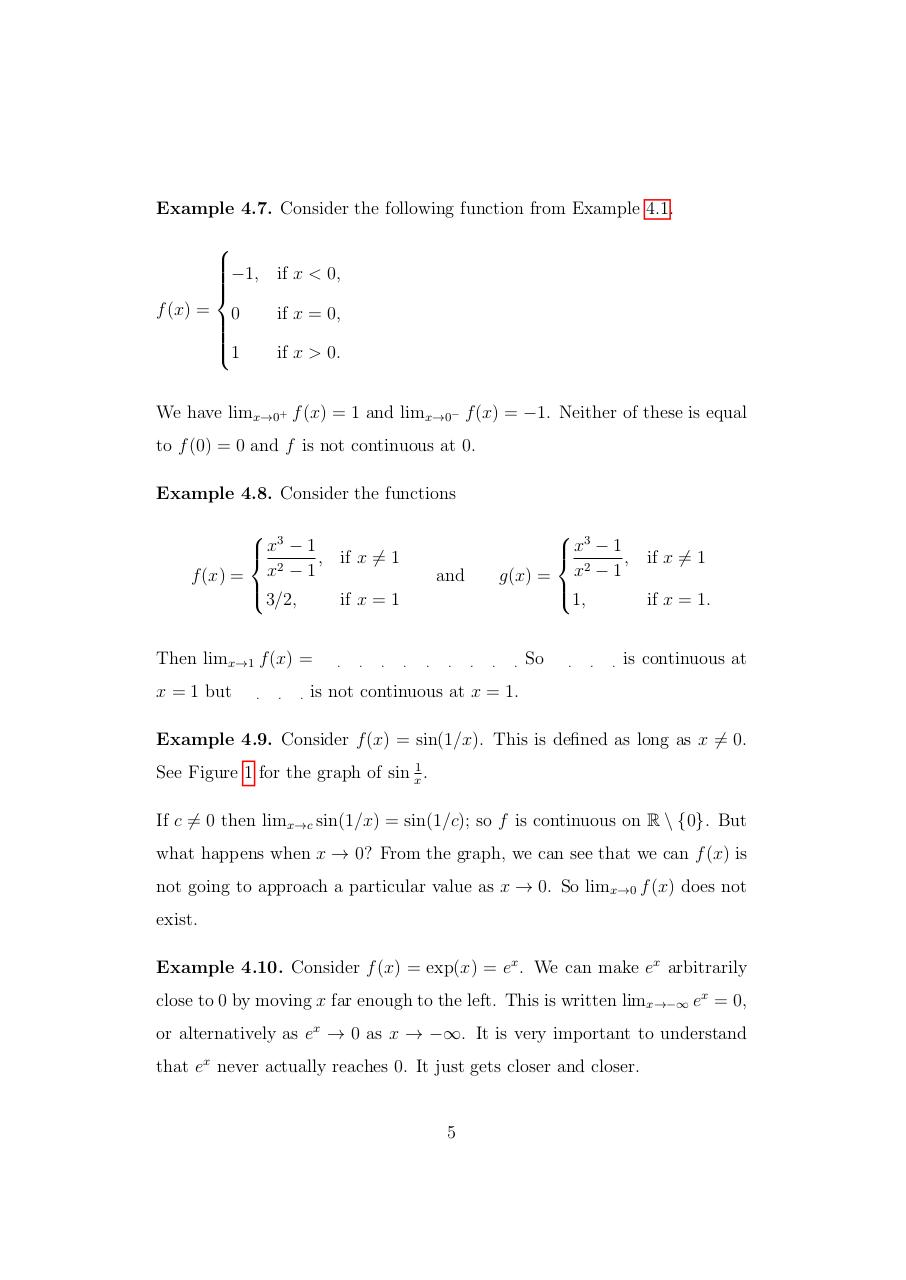# Limits.pdfPage 1 2 3 4 5 6 7 8 9 10 11 12

#### Text preview

Example 4.7. Consider the following function from Example 4.1.

−1, if x &lt; 0,

f (x) = 0
if x = 0,

1
if x &gt; 0.
We have limx→0+ f (x) = 1 and limx→0− f (x) = −1. Neither of these is equal
to f (0) = 0 and f is not continuous at 0.
Example 4.8. Consider the functions
 3

 x − 1 , if x 6= 1
2
f (x) = x − 1

3/2,
if x = 1
Then limx→1 f (x) =
x = 1 but

.

.

.

.

.

.

 3

 x − 1 , if x 6= 1
2
g(x) = x − 1

1,
if x = 1.

and

.

.

.

.

.

.

So

.

.

.

is continuous at

is not continuous at x = 1.

Example 4.9. Consider f (x) = sin(1/x). This is defined as long as x 6= 0.
See Figure 1 for the graph of sin x1 .
If c 6= 0 then limx→c sin(1/x) = sin(1/c); so f is continuous on R \ {0}. But
what happens when x → 0? From the graph, we can see that we can f (x) is
not going to approach a particular value as x → 0. So limx→0 f (x) does not
exist.
Example 4.10. Consider f (x) = exp(x) = ex . We can make ex arbitrarily
close to 0 by moving x far enough to the left. This is written limx→−∞ ex = 0,
or alternatively as ex → 0 as x → −∞. It is very important to understand
that ex never actually reaches 0. It just gets closer and closer.

5